Next: Land data example Up: Noise attenuation by filtering Previous: From filtering to covariance-pattern

# Synthetic and field data examples

Figurea displays a synthetic CMP gather composed of hyperbolas (the signal) and a monochromatic plane-wave (the noise). The true signal and noise are shown in Figuresb andc with their (f,k) spectra. It is interesting to notice that the noise and signal overlap in the (f,k) domain. This can be seen in the CMP gather where the noise has (1) similar dips to some hyperbola tails, and (2) similar frequency content. This overlap makes signal preservation particularly difficult to achieve with simple filtering techniques such as bandpass.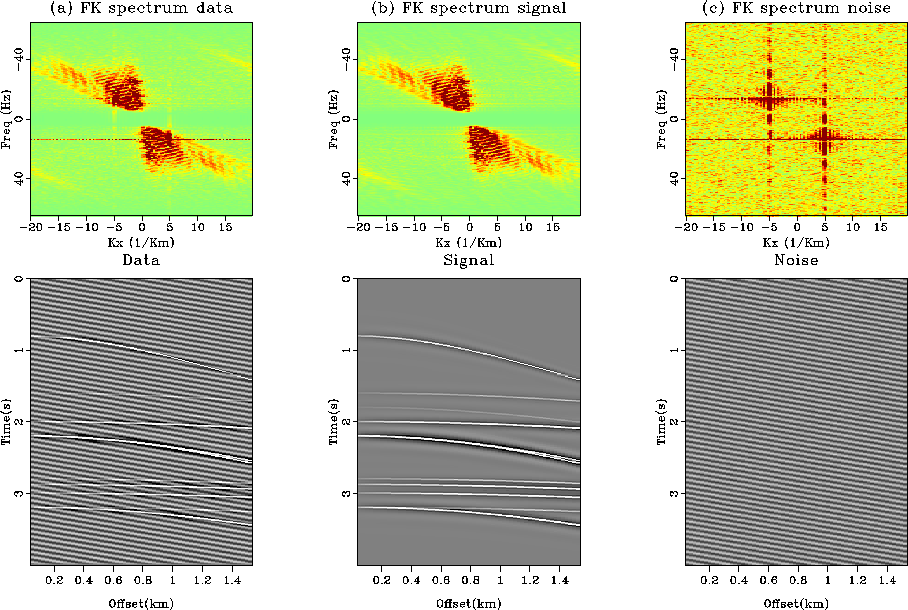datasynth
Figure 2
A synthetic data example with (a) the data and its (f,k) spectrum, (b) the true signal and its (f,k) spectrum, and (c) the noise. The spectra of signal and the noise overlap, which makes simple filtering (i.e., without inversion) likely to fail.From this dataset, the goal is to estimate in a least-squares sense a velocity panel with the hyperbolic radon transform of Chapter(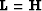). This velocity panel can be later used for velocity analysis or multiple attenuation Alvarez and Larner (2004); Foster and Mosher (1992); Thorson and Claerbout (1985). A conjugate-gradient (CG) solver is used for the iterations. Figurea illustrates the effects of the coherent noise on the model space after inversion of the data in Figurea. Some energy corresponding to both the signal and the noise is present in Figurea, but overall, it is quite difficult to recognize events corresponding to the signal. The remodeled data in Figureb (i.e.,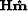) indicate that the inversion is fitting both the noise and signal, which creates artifacts in Figurea. In addition, note that the residual (i.e.,) in Figurec is not IID: a lot of coherent energy remains. Therefore, the filtering and modeling techniques are needed to obtain (1) IID residuals, (2) a better velocity panel, and (3) noise-free remodeled data.synth-nopef
Figure 3
(a) Estimated model space after inversion of the noisy data in Figurea. (b) Remodeled data from the inverted model. (c) Residual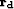after inversion. The residual is not IID and a lot of noise contaminates the model space.In this example, the noise is assumed to be known. For the filtering approach, a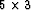PEF is used for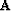in equation (). For the modeling approach,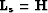and,being the same PEF that for the filtering approach.

First, as an illustration on how a simple prediction-filter differs from a projection filter, Figurea displays the spectrum of the PEFonly and Figureb the spectrum of the projection filter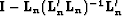with.The PEF is estimated from the known noise, and we notice that its spectrum in Figurea is the smallest at the noise location. The amplitude varies a lot with local minima and a maximum amplitude far from one, however. The spectrum of the projection filter in Figureb is better behaved: it equals zero at the noise location and is flat almost everywhere. The maximum energy is not exactly one because a damping term was added for the division.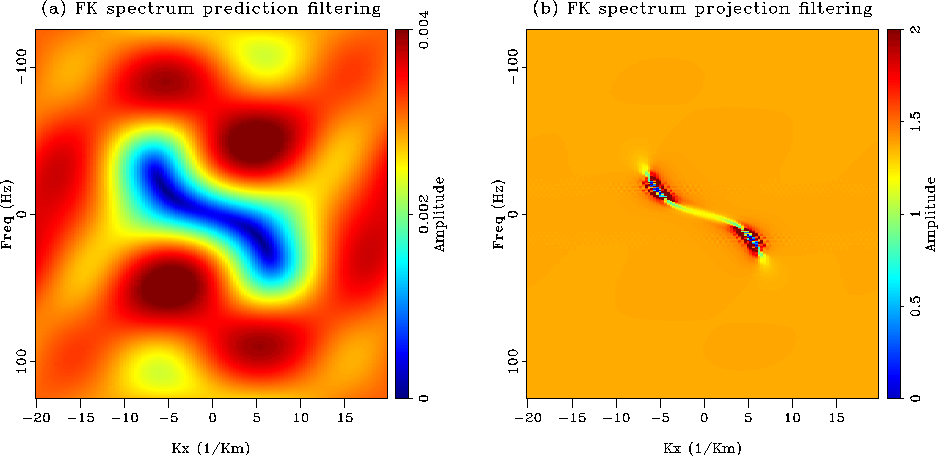pefs
Figure 4
(a) (f,k) spectrum of the PEF. It is minimum at the noise location but varies a lot everywhere else. (b) (f,k) spectrum of the projection filter. It is minimum at the noise location and flat otherwise. The projection filter is a better filter than the prediction filter.Figuredisplays the result of the inversion with the filtering approach. The estimated model in Figurea is now noise-free and the hyperbolas are well focused in the velocity space. The residual in Figured has no coherent energy left and is IID. The remodeled data in Figureb are also basically noise free, and so is the unweighted residual () in Figurec. Therefore, the filtering technique worked very well and delivered the expected results.c-synth
Figure 5
Inversion result with the filtering technique. (a) Estimated model. (b) Remodeled data. (c) Unweighted residual, which shows the coherent noise only. (d) Residual. The estimated model is artifacts free and the residual is IID. The movie shows the four panel as a function of the iterations.Figureshows the result of the modeling approach. Remember that the inverse of thePEF estimated from the true noise is used for the noise operator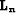and that the hyperbolic radon transform is used to model the signal. The balancing parameterin equation () is chosen by trial and error.

The estimated model after inversion is shown in Figurea. Similar to what we observed with the filtering approach, the model is noise free and easy to interpret. The remodeled data in Figureb show a little bit of coherent noise. The problem stems from the fact that the two modeling operators overlap: the inverse PEF can model some tails of hyperbolas and the radon transform can fit some of the coherent noise (because the velocity of the noise is within the range of the slowness scan). Although not crucial here, a regularization term would improve this result Nemeth (1996). The estimated noise of Figurec proves that the separation worked very well. A little bit of one hyperbola has been absorbed, however. The residual in Figured is IID which confirms that the goals of this technique were reached.compsy
Figure 6
Inversion result with the modeling technique. (a) Estimated model. (b) Remodeled data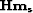.(c) Remodeled noise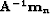.(d) Residual. The residual is IID and the model space is clean. Because the signal and noise modeling operators tend to overlap, a little bit of noise leaks in the estimated signal. This effect is very small, however.